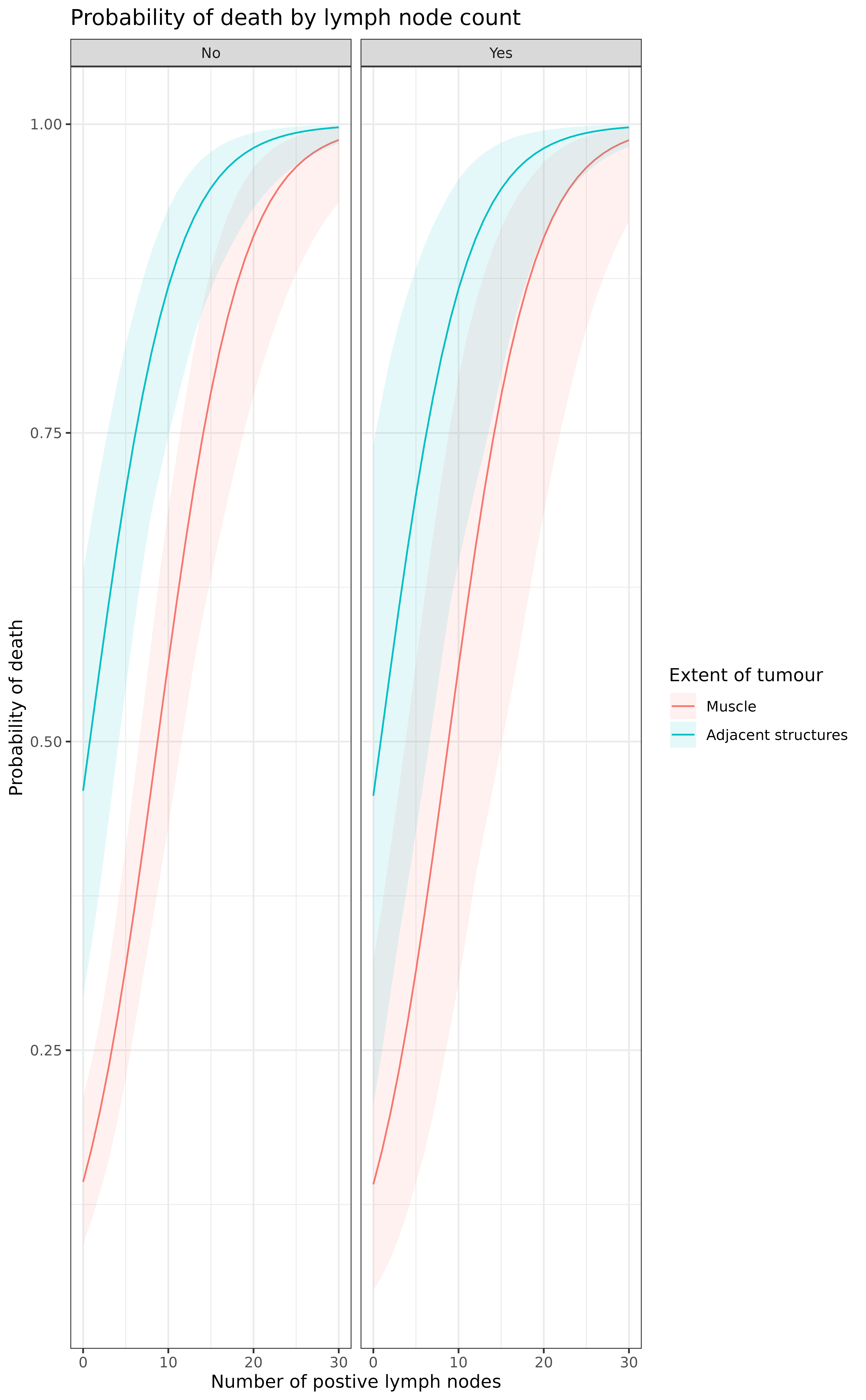Generate model predictions against a specified set of explanatory levels with bootstrapped confidence intervals. Add a comparison by difference or ratio of the first row of newdata with all subsequent rows.

boot_predict(
fit,
newdata,
type = "response",
R = 100,
estimate_name = NULL,
confint_sep = " to ",
condense = TRUE,
boot_compare = TRUE,
compare_name = NULL,
comparison = "difference",
ref_symbol = "-",
digits = c(2, 3)
)

## Arguments

fit

A model generated using lm, glm, lmmulti, and glmmulti.

newdata

Dataframe usually generated with finalfit_newdata.

type

the type of prediction required, see predict.glm. The default for glm models is on the scale of the response variable. Thus for a binomial model the default predictions are predicted probabilities.

R

Number of simulations. Note default R=100 is very low.

estimate_name

Name to be given to prediction variable y-hat.

confint_sep

String separating lower and upper confidence interval

condense

Logical. FALSE gives numeric values, usually for plotting. TRUE gives table for final output.

boot_compare

Include a comparison with the first row of newdata with all subsequent rows. See boot_compare.

compare_name

Name to be given to comparison metric.

comparison

Either "difference" or "ratio".

ref_symbol

Reference level symbol

digits

Rounding for estimate values and p-values, default c(2,3).

## Value

A dataframe of predicted values and confidence intervals, with the option of including a comparison of difference between first row and all subsequent rows of newdata.

## Details

To use this, first generate newdata for specified levels of explanatory variables using finalfit_newdata. Pass model objects from lm, glm, lmmulti, and glmmulti. The comparison metrics are made on individual bootstrap samples distribution returned as a mean with confidence intervals. A p-value is generated on the proportion of values on the other side of the null from the mean, e.g. for a ratio greater than 1.0, p is the number of bootstrapped predictions under 1.0, multiplied by two so is two-sided.

finalfit_newdata

/codefinalfit predict functions

## Examples

library(finalfit)
library(dplyr)
#>
#> Attaching package: ‘dplyr’
#> The following objects are masked from ‘package:stats’:
#>
#>     filter, lag
#> The following objects are masked from ‘package:base’:
#>
#>     intersect, setdiff, setequal, union

# Predict probability of death across combinations of factor levels
explanatory = c("age.factor", "extent.factor", "perfor.factor")
dependent = 'mort_5yr'

# Generate combination of factor levels
colon_s %>%
finalfit_newdata(explanatory = explanatory, newdata = list(
c("<40 years",  "Submucosa", "No"),
c("<40 years", "Submucosa", "Yes"),
c("<40 years", "Adjacent structures", "No"),
c("<40 years", "Adjacent structures", "Yes")
)) -> newdata

# Run simulation
colon_s %>%
glmmulti(dependent, explanatory) %>%
boot_predict(newdata, estimate_name = "Predicted probability of death",
compare_name = "Absolute risk difference", R=100, digits = c(2,3))
#>         Age    Extent of spread Perforation Predicted probability of death
#> 1 <40 years           Submucosa          No            0.28 (0.00 to 0.61)
#> 2 <40 years           Submucosa         Yes            0.29 (0.00 to 0.68)
#> 3 <40 years Adjacent structures          No            0.71 (0.55 to 0.86)
#> 4 <40 years Adjacent structures         Yes            0.72 (0.44 to 0.89)
#>        Absolute risk difference
#> 1                             -
#> 2 0.00 (-0.12 to 0.20, p=0.960)
#> 3  0.42 (0.10 to 0.64, p<0.001)
#> 4  0.40 (0.13 to 0.66, p=0.020)

# Plotting
explanatory = c("nodes", "extent.factor", "perfor.factor")
colon_s %>%
finalfit_newdata(explanatory = explanatory, rowwise = FALSE, newdata = list(
rep(seq(0, 30), 4),
c(rep("Muscle", 62), rep("Adjacent structures", 62)),
c(rep("No", 31), rep("Yes", 31), rep("No", 31), rep("Yes", 31))
)) -> newdata

colon_s %>%
glmmulti(dependent, explanatory) %>%
boot_predict(newdata, boot_compare = FALSE, R=100, condense=FALSE) -> plot

library(ggplot2)
theme_set(theme_bw())
plot %>%
ggplot(aes(x = nodes, y = estimate, ymin = estimate_conf.low,
ymax = estimate_conf.high, fill=extent.factor))+
geom_line(aes(colour = extent.factor))+
geom_ribbon(alpha=0.1)+
facet_grid(.~perfor.factor)+
xlab("Number of postive lymph nodes")+
ylab("Probability of death")+
labs(fill = "Extent of tumour", colour = "Extent of tumour")+
ggtitle("Probability of death by lymph node count")